# Introduction to Boolean Logic

• Last Updated : 26 Sep, 2021

Boolean algebra is a type of algebra that is created by operating the binary system. In the year 1954, George Boole, an English mathematician, proposed this algebra. This is a variant of Aristotle’s propositional logic that uses the symbols 0 and 1, or True and False. Boolean algebra is concerned with binary variables and logic operations.

### Boolean Expression and Variables

A Boolean expression is an expression that produces a Boolean value when evaluated, true or false, the only way to express a Boolean value. Whereas boolean variables are variables that store Boolean numbers. P + Q = R is a Boolean phrase in which P, Q, R are Boolean variables that can only store two values: 0 and 1. The computer performs all operations using binary 0 and 1 as the computer understands machine language (0/1). Boolean logic, named after George Boole, is a type of algebra in which all values are reduced to one of two possibilities: 1 or 0. To effectively comprehend Boolean logic, we must first comprehend the rules of Boolean logic, as well as the truth table and logic gates.

Attention reader! All those who say programming isn't for kids, just haven't met the right mentors yet. Join the  Demo Class for First Step to Coding Coursespecifically designed for students of class 8 to 12.

The students will get to learn more about the world of programming in these free classes which will definitely help them in making a wise career choice in the future.

### Truth Tables

A truth table represents all the variety of combinations of input values and outputs in a tabular manner. All the possibilities of the input and output are shown in it and hence the name truth table is kept. In logic problems such as Boolean algebra and electronic circuits, truth tables are commonly used. T or 1 denotes ‘True’ & F or 0 denotes ‘False’ in the truth table.

Example:

### Logic Gates

A logic gate is a virtual or physical device that performs a Boolean function. These are used to make logic circuits. Logic gates are the main components of any digital system. This electrical circuit can have only one output and 1 or more inputs. The relation between the input and the output is governed by specific logic. AND, OR, NOT gate, etc are the examples of logic gates.

### Types of Logic Gates

1. AND Gate (Product): A logic gate with two or more inputs and a single output is known as an AND gate. The logic multiplication rules are used to operate an AND gate. An AND gate can have any number of inputs, although the most common are two and three-input AND gates. If any of the inputs are low (0), the output is also low in this gate. When all of the inputs are high (1), the output will be high as well.

Logical circuit: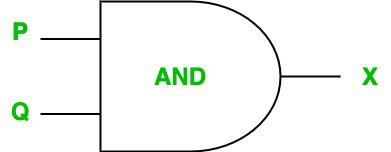Truth table:

For AND gate, the output X is true if and only if both the inputs P and Q are true. So the truth table of AND gate is as follows:

2. OR Gate (Sum): A logic gate that performs a logical OR operation is known as an OR gate. If one or both of the gate’s inputs are high, the logical OR operation produces a high output (1). (1). If neither of the inputs is high, the result is a low output (0). In the same way that an AND gate can have an unlimited number of input probes, an OR gate can only have one output probe. A logical OR gate finds the maximum between two binary digits.

Logical circuit: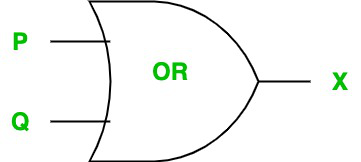Truth table:

For the OR gate, the output X is true if and only if any of the inputs P or Q is true. So the truth table of OR gate is as follows:

3. NOT Gate (Complement): Inverting NOT gates are those devices that takes only one input with an output level that is ordinarily at logic level 1 and goes low to a logic level 0 when their single input is at logic level 1, or in other words, they invert their input signal. A NOT gate’s output only returns high, when its input is at logic level 0. The output ~P (~ denotes Not) of a single input NOT gate is only true when the input P is false or we can say, Not true. It is also called inverse gate as it results the negation of the input Boolean Expression.

Logical circuit: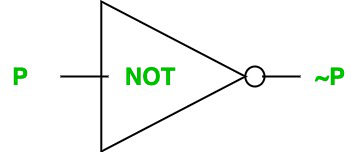Truth table:

For the NOT gate, the output X is true if and only if input P is false. So the truth table of NOT gate is as follows:

4. NAND Gate: A logic gate known as a NAND gate provides a low output (0) only if all of its inputs are true, and high output (1) otherwise. As a result, the NAND gate is the inverse of an AND gate, and its circuit is created by joining AND gate and NOT gate. NAND means ‘Not of AND’ Gate and it results in false only when both the inputs P and Q are true. AND gates (together with NOR gates) are known as universal gates because they are a form of logic gate that can implement any Boolean function without the usage of any other gate type.

Logical circuit: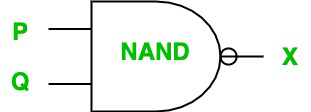Truth table:

For the NAND gate, the output X is false if and only if both the inputs(i.e., P and Q) are true. So the truth table of the NAND gate is as follows:

5. NOR Gate: A logic gate known as a NOR gate provides a high output (1) only if all of its inputs are false, and low output (0) otherwise. As a result, the NOR gate is the inverse of an OR gate, and its circuit is created by joining OR gate and NOT gate. NOR means ‘Not of OR’ Gate & it results in true only when both the inputs P and Q are false.

Logical circuit: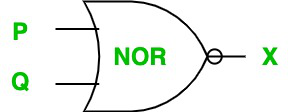Truth table:

For the NAND gate, the output X is true if and only if both the inputs(i.e., P and Q) are false. So the truth table of NOR gate is as follows:

6. XOR Gate: An XOR gate (also known as an Exclusive OR gate) is a digital logic gate that conducts exclusive disjunction and has two or more inputs and one output. Only one of an XOR gate’s inputs must be true for the output to be true. The output of an XOR gate is false if both of its inputs are false, or true if both of its inputs are true. XOR means ‘Exclusive OR’ Gate & it results in true only when either of the 2 inputs P & Q is true, i.e., either P is true or Q is true but not both.

Logical circuit: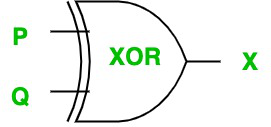Truth table:

7. XNOR Gate: An NOR gate (also known as an Exclusive NOR gate) is a digital logic gate that is just opposite of XOR gate. It has two or more inputs and one output. When one of its two input is true but not both then it will return false. XNOR means ‘Exclusive NOR’ Gate and it result is true only when both of its inputs P and Q are either true or false.

Logical circuit: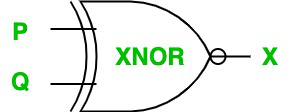Truth table:

### Laws for Boolean Logic

Following are some laws for boolean logic:

### De Morgan’s laws

De Morgan’s Law states that:

Statement 1: The Complement of the product (AND) of two Boolean variables (or expressions) is equal to the sum(OR) of the complement of each Boolean variable (or expression).

~(P.Q) = (~P) + (~Q)

Proof:

Statement: ~(P.Q) = (~P) + (~Q)

The truth table is:

We can clearly see that truth values for ~(P.Q) are equal to truth values for (~P) + (~Q), corresponding to the same input.

Statement 2: The Complement of sum (OR) of two Boolean variables (or expressions) is equal to the product(AND) of the complement of each Boolean variable (or expression).

~(P + Q) = (~P).(~Q)

Proof

Statement: ~(P+Q) = (~P).(~Q)

The truth table is :

We can clearly see that truth values for ~(P + Q) are equal to truth values for (~P).(~Q), corresponding to the same input.

### Logic circuits

An electric circuit in which we can give one or more binary inputs (assuming two states, on or off) and we get a single binary output corresponding to the input in a fashion that can be described as a function in symbolic logic. The AND, OR, and NOT gates are basic logic circuits that perform the logical functions – AND, OR, and NOT, respectively. Computers can do more complicated tasks with circuits than they could with only a single gate.

Example: A chain of two logic gates is the smallest circuit. Consider the following circuit: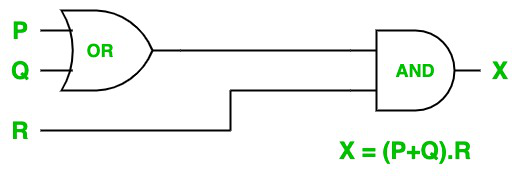This logic circuit is for the Boolean expression : (P + Q).R.

Here, the first OR gate is used : P, Q are input to it and P + Q is the output.

Then, AND gate is used : (P + Q), R is input to it & (P + Q).R is the output.

So the truth table is :

### Sample Questions

Question 1. What are universal gates?

Solution:

Universal gates are logic gates that can implement any Boolean function without necessitating the use of any additional gates. There are just two universal gates in digital electronics:
1. NAND Gate and
2. NOR Gate.

Question 2. Design the logical circuit for: A.B + B.C

Solution: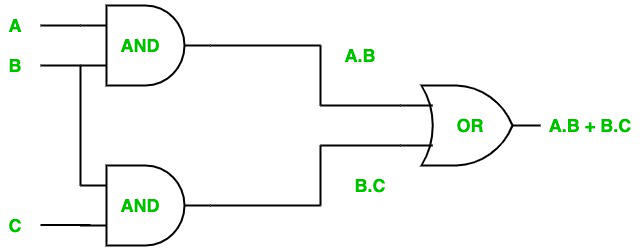Question 3. What will be the Boolean expression for the following logic circuit :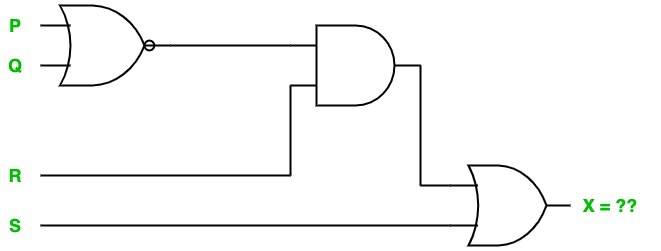Solution:

X = ~(P + Q).R + S

Question 4. Verify using truth table: P + P.Q = P

Solution:

The truth table for P + P.Q = P

In the truth table, we can see that the truth values for P + P.Q is exactly the same as P.

My Personal Notes arrow_drop_up